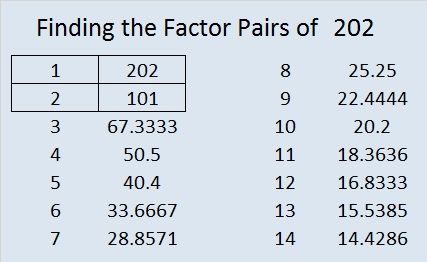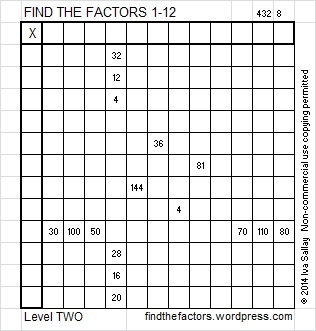# 202 and Level 2

• 202 is a composite number.
• Prime factorization: 202 = 2 x 101
• The exponents in the prime factorization are 1 and 1. Adding one to each and multiplying we get (1 + 1)(1 + 1) = 2 x 2 = 4. Therefore 202 has exactly 4 factors.
• Factors of 202: 1, 2, 101, 202
• Factor pairs: 202 = 1 x 202 or 2 x 101
• 202 has no square factors that allow its square root to be simplified. √202 ≈ 14.2126704——————————–Excel file of puzzles and previous week’s factor solutions: 12 Factors 2014-08-11